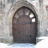Choose Language Hide Translation Bar
Highlighted

## Using Hough Transform

Hi,

I want to use the Hough tranform function in a program I am writing to detect edges, however I am a little confused by what values the input matrix should contain. I tried using the scripting index but it wasn't particularly helpful. Is the matrix the matrix of rgb values, hsl values, or a column vector of intensity values? Thanks!

Noah

4 REPLIES 4
Highlightedsusan_walsh1
Staff (Retired)

## Re: Using Hough Transform

Hi, Noah.

The JSL Syntax Reference has a little more information on this function than the Scripting Index. There is says that "it takes a matrix of intensities and transforms it in a way that is useful for finding streaks in the matrix."

Highlighted

## Re: Using Hough Transform

Not answering your question, but I did find this explanation of the transform

Craige
Highlighted

## Re: Using Hough Transform

Here's what I've learned:

the input matrix is an approximately square matrix of non-negative values that are accumulated into the output using the transform. I believe the transform is actually the sin/cos variation described at the end of the video. There are two parameters you can use in the function call:

HoughLineTransform( M, NAngle(180), NRadius( r) )

specified by name, after the matrix. 180 is the default number of angles and is the number of columns in the output matrix. "r" defaults to sqrt(nr^2+nc^2), roughly the diagonal "pixels" of the matrix, and is the number of rows in the output matrix.

I'd also suggest looking at the canny filter in the scripting index; it has a very cool example (2nd example under filter).Canny filter example from scripting index

Craige
Highlighted

## Re: Using Hough Transform

Blog post

Craige
Article Labels

There are no labels assigned to this post.